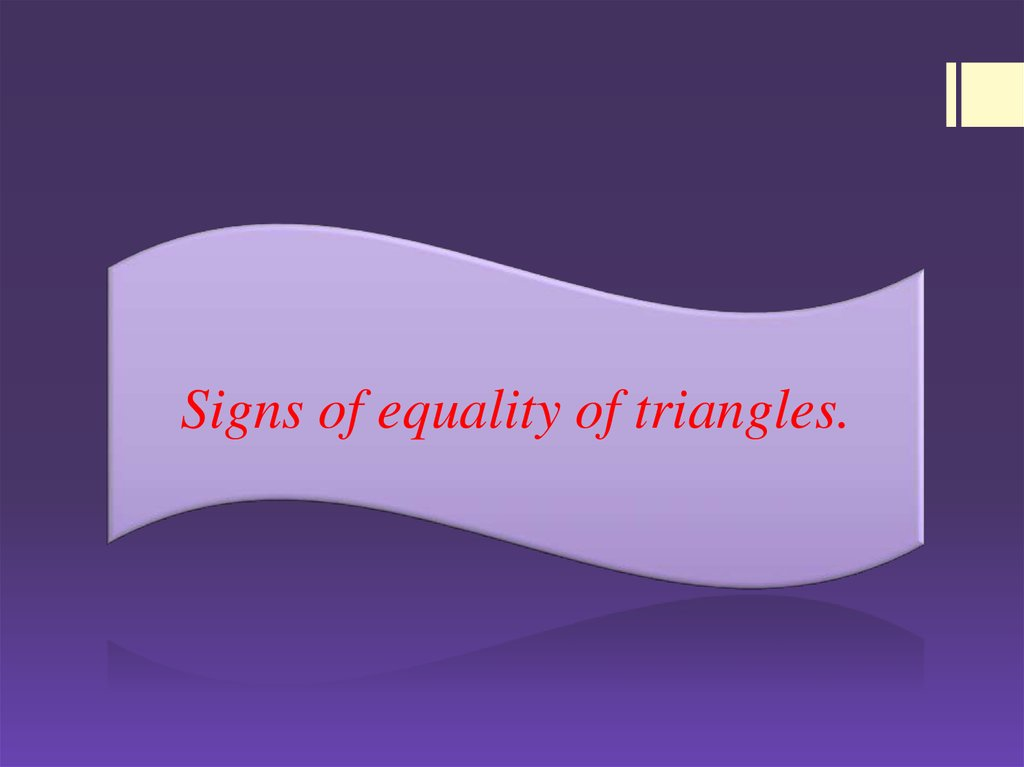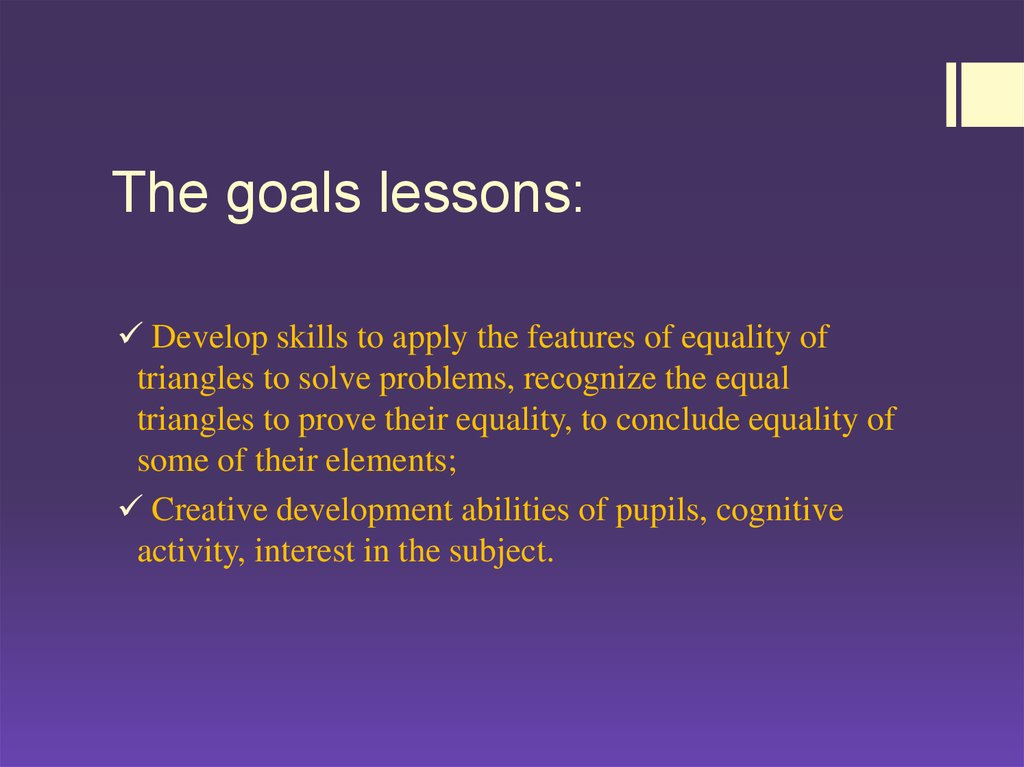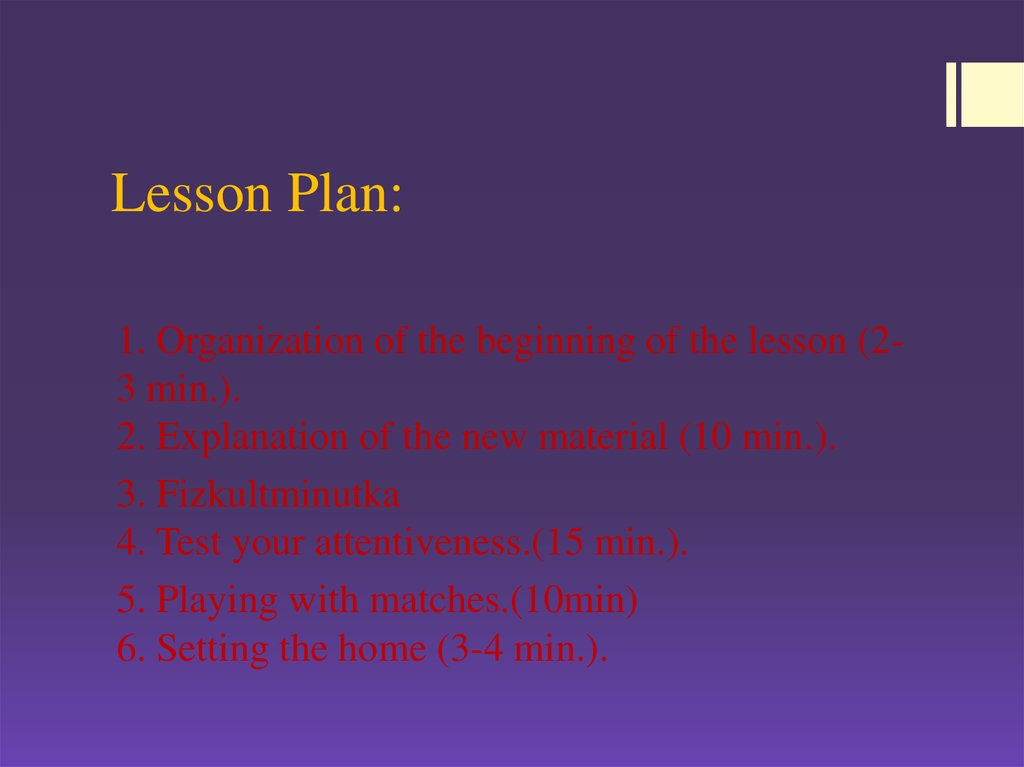# Sign of equality of triangles. lesson 2

## 1.

Signs of equality of triangles.

## 2. The goals lessons:

Develop skills to apply the features of equality of
triangles to solve problems, recognize the equal
triangles to prove their equality, to conclude equality of
some of their elements;
Creative development abilities of pupils, cognitive
activity, interest in the subject.

## 3. Lesson Plan:

1. Organization of the beginning of the lesson (23 min.).
2. Explanation of the new material (10 min.).
3. Fizkultminutka
5. Playing with matches.(10min)
6. Setting the home (3-4 min.).

## 7. 4. Fizkultminutka

4.1 Inhale breath, stretched.
4.2 Hands - up, worked her fingers - to make different
triangles.
4.3 With his left hand in the air to draw a triangle, then - right,
and - two.
4.4 In tandem with the neighbor (I have two hands - the two
sides, a neighbor helps make a triangle).
4.5 Draw a triangle on the floor of each leg.
4.6 shook off fatigue with hands, feet. Sit down.

## 8.

Pick triangles equal data. Place them in the same order.

## 9.

Pick triangles equal data. Place them in the same order.

## 10. 1. Of the five matches be 2 equilateral triangles. 2. Of the six matches to make four equilateral triangles.

1. Put three matches so as to obtain five equal triangles(For example)

1. HOMEWORK
Page: 92-93
Exercise №5,7,14

## 12.

AN UNUSUAL TRIANGLE
Triangle divided into 4 parts, and then make a triangle are
equal to the first, but with an empty square. What's the
secret? To square disappeared?
(Треугольник разделили на 4 части, а потом из них составили
треугольник, равный первому, но с пустым квадратом. В чем
секрет? Куда исчез квадрат?)